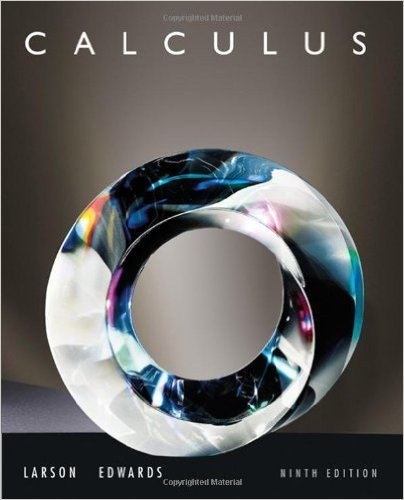×
×

# Solutions for Chapter 7.7: Fluid Pressure and Fluid Force## Full solutions for Calculus | 9th Edition

ISBN: 9780547167022Solutions for Chapter 7.7: Fluid Pressure and Fluid Force

Solutions for Chapter 7.7
4 5 0 248 Reviews
28
3
##### ISBN: 9780547167022

Since 36 problems in chapter 7.7: Fluid Pressure and Fluid Force have been answered, more than 67456 students have viewed full step-by-step solutions from this chapter. This expansive textbook survival guide covers the following chapters and their solutions. This textbook survival guide was created for the textbook: Calculus , edition: 9. Calculus was written by and is associated to the ISBN: 9780547167022. Chapter 7.7: Fluid Pressure and Fluid Force includes 36 full step-by-step solutions.

Key Calculus Terms and definitions covered in this textbook
• Deductive reasoning

The process of utilizing general information to prove a specific hypothesis

• Difference of functions

(ƒ - g)(x) = ƒ(x) - g(x)

• Double inequality

A statement that describes a bounded interval, such as 3 ? x < 5

• Focal axis

The line through the focus and perpendicular to the directrix of a conic.

See Polynomial function in x

• Leibniz notation

The notation dy/dx for the derivative of ƒ.

• Line of travel

The path along which an object travels

• Magnitude of a vector

The magnitude of <a, b> is 2a2 + b2. The magnitude of <a, b, c> is 2a2 + b2 + c2

• Matrix element

Any of the real numbers in a matrix

• Negative angle

Angle generated by clockwise rotation.

• Parametric curve

The graph of parametric equations.

• Parametric equations for a line in space

The line through P0(x 0, y0, z 0) in the direction of the nonzero vector v = <a, b, c> has parametric equations x = x 0 + at, y = y 0 + bt, z = z0 + ct.

• Random behavior

Behavior that is determined only by the laws of probability.

• Range of a function

The set of all output values corresponding to elements in the domain.

• Recursively defined sequence

A sequence defined by giving the first term (or the first few terms) along with a procedure for finding the subsequent terms.

• Second quartile

See Quartile.

• Semimajor axis

The distance from the center to a vertex of an ellipse.

• Variable (in statistics)

A characteristic of individuals that is being identified or measured.

• Vertex of a parabola

The point of intersection of a parabola and its line of symmetry.

• Wrapping function

The function that associates points on the unit circle with points on the real number line

×